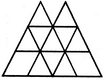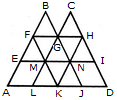# Non Verbal Reasoning - Analytical Reasoning - Discussion

Discussion Forum : Analytical Reasoning - Section 1 (Q.No. 21)
21.

Find the number of triangles in the given figure.16
18
14
15
Explanation:

The figure may be labelled as shown.The simplest triangles are BFG, CGH, EFM, FMG, GMN, GHN, HNI, LMK, MNK and KNJ i.e. 10 in number.

The triangles composed of three components each are FAK and HKD i.e. 2 in number.

The triangles composed of four components each are BEN, CMI, GLJ and FHK i.e. 4 in number.

The triangles composed of eight components each are BAJ and OLD i.e. 2 in number.

Thus, there are 10 + 2 + 4 + 2 = 18 triangles in the given figure.

Discussion:
1 comments Page 1 of 1.

Jasmin baral said:   2 years ago
Explain me to get it clearly.